# Perron method

A method for solving the Dirichlet problem for the Laplace equation based on the properties of subharmonic functions (and superharmonic functions, cf. Subharmonic function). O. Perron  gave the initial presentation of the method, which was substantially developed by N. Wiener

and M.V. Keldysh .

Letbe a bounded domain in a Euclidean space,, with boundary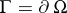, let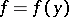be a real-valued function on,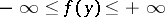. Letbe the non-empty family of all superharmonic functions,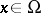, in the wide sense (i.e. the function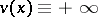belongs to) that are bounded from below and are such that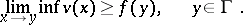Letbe the lower envelope of. Along with, consider the non-empty familyof all subharmonic functions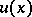,, in the wide sense (the function) that are bounded from above and are such thatLetbe the upper envelope of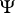.

There are only three possibilities for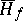(and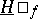):,or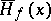is a harmonic function; and alwaysThe function,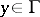, is called resolutive if the two envelopesandare finite and coincide. In that case the harmonic function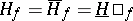is the generalized solution to the Dirichlet problem for the function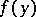,(in the sense of Wiener–Perron). For,, to be resolutive it is necessary and sufficient that it be integrable with respect to the harmonic measure on(Brelot's theorem). Any continuous finite function,, is resolutive (Wiener's theorem).

A point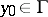is called a regular boundary point if the following limit relation applies for any continuous finite function,: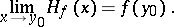Regularity at all pointsis equivalent to the existence of classical solutions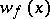to the Dirichlet problem for any continuous finite function,, and in that case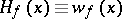; a bounded domainall boundary points of which are regular is sometimes also called regular. For a pointto be regular it is necessary and sufficient that there is a barrier at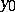.

Pointsthat are not regular are called irregular boundary points. For example, isolated points are irregular boundary points, as are the vertices of sufficiently sharp wedges enteringif(Lebesgue spines). The set of all irregular points ofis a set of type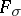of capacity zero.

Let there be a sequence of domains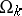,, such that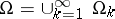, and let a continuous finite function,, be continuously extendible to. Then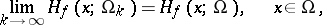uniformly on compact sets in; in the case of regular domainsone obtains a construction à la Wiener for the generalized solution to the Dirichlet problem. Now consider an arbitrary sequence of domains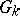,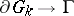,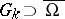, for a domainwithout an interior boundary. In that case, in general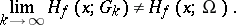The Dirichlet problem is stable in a domainor in a closed domainif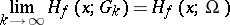for allor for all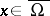, respectively. For the Dirichlet problem to be stable in a domainit is necessary and sufficient that the sets of all irregular points in the complements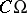andcoincide; stability in a closed domain requires thatdoes not have irregular points (Keldysh' theorems, cf. Keldysh theorem and , where an example is also constructed of a regular domainwithin which the Dirichlet problem is unstable).